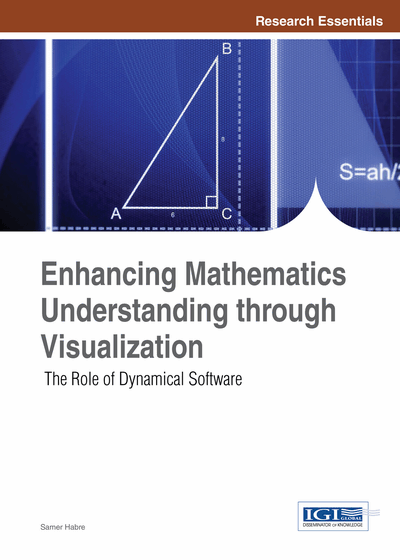# Technology and Differential Equations

John Hubbard (Cornell University, USA)
DOI: 10.4018/978-1-4666-4050-4.ch001
Available
\$37.50
No Current Special Offers

## Abstract

Differential equations are the main way in which we make mathematical models of the real world. This is true in just about all fields, from physics to fluid mechanics, from astronomy to finance: if you want to understand how something evolves, or if you want to understand equilibria, you will need to set up and “solve” differential equations. For instance, our understanding of global warming depends mainly on analyzing the differential equations modeling the weather and seeing how their behavior depends on the concentration of greenhouse gasses in the atmosphere. The future of humanity depends on our getting it right.
Chapter Preview
Top

## The Standard Curriculum

This grandiose view of the central importance of differential equations in how we understand the world is not much in evidence in courses on differential equations, particularly not in elementary courses.

### The First Course

The students are likely to encounter a bag of tricks to find solutions to various equations, especially first order separable equations and second-order linear differential equations with constant coefficients. These are of course important topics. An explicit solution is a wonderful thing, especially if the formula is analyzed and graphed to emphasize the behavior of the solution. For linear differential equations with constant coefficients, understanding how the signs of the real parts of eigenvalues affect the behavior of solutions is critical in many situations.

I fear that often far less attention is focused on the meaning of differential equations than on obtaining the formulas.

### A More Ambitious Course

In a more ambitious first course, the students may well see in addition a proof of the existence and uniqueness theorem, probably based on Picard iteration, and solutions of the heat equation and the wave equation based on Fourier series, using separation of variables. There might also be an introduction to numerical methods, usually independent of the existence and uniqueness theory.

Again, these are important topics; but I have reservations about how they are taught in practice. The Fourier solutions to the heat and wave equations are really the first chapter of the spectral theory of differential operators, and the fact that separation of variables leads to eigenvalues and eigenfunctions, closely related to linear equations with constant coefficients, is often overlooked.

My reservations about existence and uniqueness are of a different nature. They are based on a very disturbing observation: I often had the experience of asking students questions like “does the solution ofwithexist for all t ≥ 0?” The students would fiddle with the equation for a while, try various changes of variables, and eventually come up with the conclusion that “this equation has no solutions.” These are students who could probably state the existence and uniqueness theorem, and perhaps prove it. But the “solution” provided as a limit of Picard iterations did not have the necessary psychological reality in the student’s minds for them to think about it effectively.

Top

## A More Appropriate Approach

With Beverly West I developed during the 1980’s and 90’s a different curriculum, embodied in the books Differential Equations, A Dynamical Systems Approach, Parts I and II (Hubbard & West, 1991, 1995), and the software package MacMath (Hubbard & West, 1992, 1999)

• Principle 1: Solutions to differential equations are functions, and the object of a course on differential equations is to describe the behavior of such functions.

By behavior, I mean answers to such questions as:

• 1.

Determine or approximate the domain of existence of solutions.

• 2.

How do the solutions behave at the boundary of the domain of existence: Do they have vertical asymptotes, do they oscillate, etc.?

• 3.

Where are they increasing or decreasing? Where do they have maxima and minima?

The solutions are usually not expressible in elementary terms or as indefinite integrals of elementary functions, and the course should provide tools to describe the behavior of solutions without finding explicit solutions.

## Complete Chapter List

Search this Book:
Reset